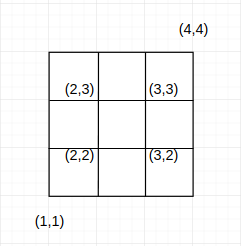InterviewBit Academy is now Scaler Academy!# The square game

You are given a square with sides parallel to the x and y axes.

You will be given the coordinates of the lower left and the
upper right vertices of the square. You need to count the number of integral points that are strictly inside the square.
The points that lie on the square should not be counted.

Image for sample case:Constraints:

``````1 <= A, B, C, D <= 1000
A != C
``````

Input:

``````An integer A corresponding to the x coordinate of the lower left vertex.
An integer B corresponding to the y coordinate of the lower left vertex.
An integer C corresponding to the x coordinate of the upper right vertex.
An integer D corresponding to the y coordinate of the upper right vertex.
``````

Output:

``````One integer corresponding to the number of integral points strictly inside the given square.
``````

Examples:

Input:

``````1
1
4
4
``````

Output:

``````4
``````

Explanation:

``````(2,3),(3,2),(2,2),(3,3) are the integral points that are strictly inside the given square. Refer the diagram given in the given question for more details.
``````
NOTE: You only need to implement the given function. Do not read input, instead use the arguments to the function. Do not print the output, instead return values as specified. Still have a doubt? Checkout Sample Codes for more details.